# Three-dimensional biological tissue under high-order effect of two-temperature thermal lagging to thermal responses due to a laser irradiation

## Hamdy M. Youssef1, Najat A. Alghamdi2

1, 2Umm Al-Qura University, Makkah, Saudi Arabia

1Corresponding author

Vibroengineering PROCEDIA, Vol. 22, 2019, p. 112-117. https://doi.org/10.21595/vp.2019.20521
Received 14 January 2019; accepted 21 January 2019; published 15 March 2019

36th International Conference on Vibroengineering in Dubai, United Arab Emirates, March 15-17th, 2019

Copyright © 2019 Hamdy M. Youssef, et al. This is an open access article distributed under the Creative Commons Attribution License, which permits unrestricted use, distribution, and reproduction in any medium, provided the original work is properly cited.
Views 80
Abstract.

Using the lasers and heat transfer through biological skin tissue is essential for the processes of the medical treatment. Thus, two-temperature bio-heat transfer equations were introduced and used to discuss the variation of temperature in a laser-irradiated biological tissue. As a result, the Laplace and Fourier transform techniques have been applied, and the solutions of the conductive temperature have been represented in figures. The two-temperature parameter, the penetration depth parameter, the dimensions of the rectangular laser pulse, and the power density of laser irradiation parameter have significant effects on the thermal waves through the skin tissue.

Keywords: two-temperature, thermal lagging, biological tissue, laser irradiation.

#### 1. Introduction

In the medical applications, the use of lasers has increased over the past half-century. For increasing the therapy quality, the observation of thermal damage is important. Effect of the laser irradiation on the biological tissues takes different types. The most common of those applications include the thermal effects. The thermal interaction is the most important since temperature rising due to thermal effects is a final factor to produce thermal damage. Due to lack of knowledge about the thermal reaction and response, the clinical doctors could not control the times of using the laser power [1-4]. To predict the thermal damage quantity, it is required to study the thermal response caused by the laser energy deposition. As a result, the analysis of thermal transport is essential to make an improvement to the treatment qualification and for more safety. The experiment is an essential method to know the medical treatment problems [4, 5]. The most famous researchers studied the bioheat transfer in the biological skin tissues are Pennes , Abramson . The Pennes bio-heat transfer equation is used to simulate and model the thermal behavior in the biological bodies. The review paper  represented many attempts to model the thermal behavior of the human body. Xu et al. [9, 10] presented a system discussion to the DPL effects on the bio-thermo-mechanical behavior of skin tissues. The linear DPL equation has been used in various theoretical studies [11-13]. Youssef applies the two-temperature heat conduction in many applications [14, 15].

#### 2. Materials and method

Consider homogeneous skin tissue in three-dimensional fills the region $\mathrm{\Psi }$which is defined by where the skin is initially at rest and has been thermally shocked by laser irradiation with a rectangular pulse at the bounding plane to the surface. Tzou proposed the DPL model in the classical heat flux model as . Youssef proposed the two-temperature model to differentiate between the conductive temperature and the dynamical temperature as .

(1)

where $T$ is the temperature, $K$ the heat conductivity, $t$ is the time, ${\tau }_{q}$, ${\tau }_{T}$ are the phase lag of the heat flux and the phase lag of the temperature gradient, respectively, $\rho$ is the density, $C$ is the specific heat, ${C}_{b}$ and ${W}_{b}$ are the specific heat and perfusion rate of blood. ${Q}_{met}$ is the metabolic heat generation and ${Q}_{ext}$ is the heat source for spatial heating, ${T}_{b}$ is the arterial temperature, ${T}_{C}$ is the conductive temperature, and ${T}_{D}$ is the dynamical temperature:

(2)

where $\beta$ is a non-negative parameter which is called two-temperature parameter.

The term ${W}_{b}{C}_{b}\left({T}_{D}-{T}_{b}\right)$ expresses the heat caused by convection within the head per unit mass of the tissue and it is considered to be homogenous.

Assume the functions $\phi$ and $\theta$ is as follows:

(3)

From Eqs. (1) and (2), we obtain:

(4)
(5)

where ${\nabla }^{2}=\left(\frac{{\partial }^{2}}{\partial {x}^{2}}+\frac{{\partial }^{2}}{\partial {y}^{2}}+\frac{{\partial }^{2}}{\partial {z}^{2}}\right)$.

Due to the chemical reactions within the tissues, the metabolic heat source is valid, and it is assumed to be a constant ${Q}_{met}=$ 368.1 W/m3. When the laser beam shocked the skin surface, the laser energy is absorbed, scattered, and transmitted. Lambert expresses the laser power intensity along the tissue depth-Beer’s law, as follows :

(6)
$I\left(x,y,z,t\right)={I}_{0}{e}^{-\delta x}H\left(t\right)H\left(a-\left|y\right|\right)H\left(b-\left|z\right|\right)$.

Heat generation due to scattering is assumed to be negligible; therefore, the specific absorption rate in the target zone can be expressed as follows:

(7)
$-\frac{\partial I}{\partial x}={Q}_{ext}\left(x,y,z,t\right)=\mathcal{l}{I}_{0}{e}^{-\delta x}H\left(t\right)H\left(a-\left|y\right|\right)H\left(b-\left|z\right|\right).$

In the Eq. (6), ${I}_{0}$ (W/m2) represents the power density of laser irradiation and $H\left(t\right)$ is the Heaviside unit step function. The parameter $\delta$ represents the penetration depth. It means, the rectangular thermal pulse of the laser irradiation acts on a band of width 2$a$ centered on the $y$-axis and 2$b$ centered around the $z$-axis on the surface of the half-space $x=\text{0}$ and is zero elsewhere:

(8)

Applying the Laplace transform for Eqs. (5), (7) and (8) defined as:

(9)
$\stackrel{-}{f}\left(s\right)={\int }_{0}^{\mathrm{\infty }}f\left(t\right){e}^{-st}dt,$

where:

(10)
${\phi \left(x,t\right)|}_{t=0}={\theta \left(x,t\right)|}_{t=0}={\frac{\partial \phi \left(x,t\right)}{\partial t}|}_{t=0}={\frac{\partial \theta \left(x,t\right)}{\partial t}|}_{t=0}=0.$

Thus, we get:

(11)
(12)

where ${h}_{T}=\left(1+s{\tau }_{T}-\frac{{s}^{2}{\tau }_{T}^{2}}{2}\right)$, ${h}_{q}=\left(1+s{\tau }_{q}-\frac{{s}^{2}{\tau }_{q}^{2}}{2}\right)$.

#### 3. The governing equations in the Fourier transform domain

Using double Fourier transform for any function $f\left(x,y\right)$ which is defined as follows:

(20)

where the inversion transform of the double Fourier transform takes the form:

(21)

Hence, we have:

(23)

(24)
$\stackrel{~}{\stackrel{-}{\theta }}=\left[1+\beta \left({p}^{2}+{q}^{2}\right)\right]\stackrel{~}{\stackrel{-}{\phi }}-\beta \frac{{\partial }^{2}\stackrel{~}{\stackrel{-}{\phi }}}{\partial {x}^{2}}.$

By eliminating $\stackrel{~}{\stackrel{-}{\theta }}$ from Eqs. (15) and (16), we get the following differential equation:

(25)

where:

${\lambda }^{2}=\frac{{h}_{q}\left(\rho Cs+{W}_{b}{C}_{b}{\rho }_{p}\right)\left(1+\beta \left({p}^{2}+{q}^{2}\right)\right)+K{h}_{T}\left({p}^{2}+{q}^{2}\right)}{\left[K{h}_{T}+\beta {h}_{q}\left(\rho Cs+{W}_{b}{C}_{b}{\rho }_{p}\right)\right]},$

The general solution of the differential Eq. (17) takes the form:

(26)

where ${c}_{1}$ and ${c}_{2}$ are some parameters which have to be determined.

Apply the boundary conditions:

(27)

Hence, we obtain:

(28)

where:

#### 4. Results

The values of the relevant thermal parameters used in the present calculations are as follows in Table 1 [4, 9, 13, 18].

Table 1. The material properties of the skin tissue

 Parameter Unit Skin tissue Parameter Unit Skin tissue $K$ W/m°C 0.628 ${W}_{b}$ ml/Cm 0.00187 $\rho$ kg/m3 1000 ${T}_{b}$ °C 37 ${\rho }_{b}$ kg/m3 1060 ${\tau }_{T}$ s 10 $C$ J/kg°C 4187 ${\tau }_{q}$ s 15 ${C}_{b}$ J/kg°C 3860 $L$ m 0.05

#### 5. Discussion

Fig. 1 shows the conductive temperature increment with a range of $x$-axis and various positions of $y$ and $z$. The values of both $y$ and $z$ have significant effects. When the values of $y$ and $z$ are increasing, the value of the conductive temperature increment is decreasing. Fig. 2 shows the conductive temperature increment with a range of $x$-axis and various value of $\beta$ and $y=z=$ 0.0. The value of $\beta$ has a significant effect. When the value of $\beta$ is increasing, the value of the conductive temperature is increasing. Fig. 3 shows the conductive temperature distribution with a range of $x$-axis and various value of $\delta$ when $y=z=$ 0.0. The value of $\delta$ has a significant effect. When $\delta$ is decreasing, the value of the conductive temperature is decreasing. Fig. 4 shows the conductive temperature distribution with a range of $x$-axis and various $a$, $b$ and $y=z=$ 0.0. The values of $a$ and have significant effects. When the values of $a$ and are increasing, the value of the conductive temperature is increasing. Fig. 5 shows the conductive temperature distribution with a range of $x$-axis and various value of ${I}_{0}$ when $y=z=$ 0.0. The value of ${I}_{0}$ has a significant effect. When the value of ${I}_{0}$ is increasing, the value of the conductive temperature is increasing.

Fig. 1. The conductive temperature increment with various of $y$, $z$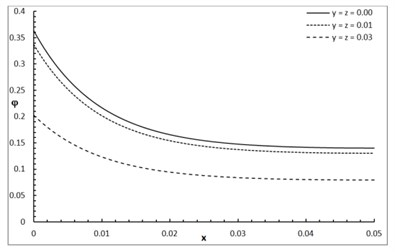Fig. 2. The conductive temperature increment distribution with various values of $\beta$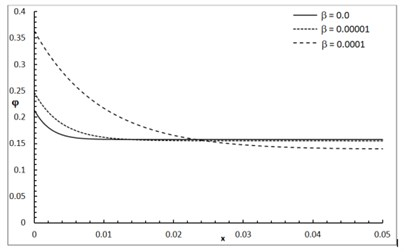Fig. 3. The conductive temperature increment distribution with various values of $\delta$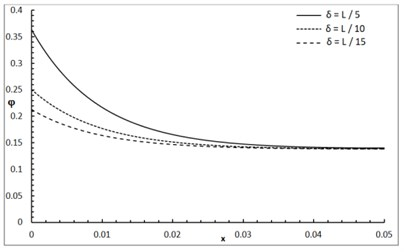Fig. 4. The conductive temperature increment distribution with various of $a$ and $b$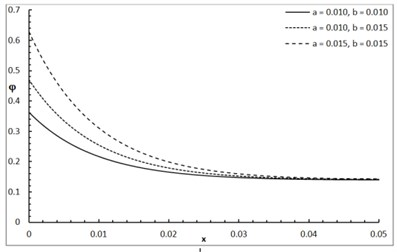Fig. 5. The conductive temperature increment with various of ${I}_{0}$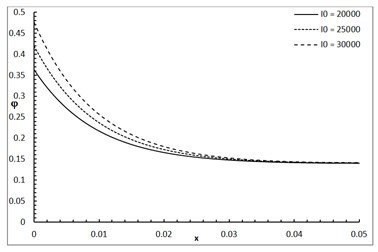#### 6. Conclusions

The two-temperature parameter, the dimensions of the rectangular laser pulse, the penetration depth parameter, and the power density of laser irradiation have significant effects on the conductive temperature.

#### Acknowledgements

The authors are grateful for the support of this work as a part of the project provided by the “Long-Term Comprehensive National Plan for Science, Technology, and Innovation” through STU of Umm Al-Qura University by Grant Number 14-MAT162-10.

1. Liu K.-C., Wang J.-C. Analysis of thermal damage to laser irradiated tissue based on the dual-phase-lag model. International Journal of Heat and Mass Transfer, Vol. 70, 2014, p. 621-628. [Publisher]
2. Welch A. The thermal response of laser irradiated tissue. IEEE Journal of Quantum Electronics, Vol. 20, Issue 12, 1984, p. 1471-1481. [Publisher]
3. Díaz S. H., Nelson J. S., Wong B. J. Rate process analysis of thermal damage in cartilage. Physics in Medicine and Biology, Vol. 48, Issue 1, 2002, p. 19. [Publisher]
4. Zhou J., Chen J., Zhang Y. Theoretical analysis of thermal damage in biological tissues caused by laser irradiation. Molecular and Cellular Biomechanics, Vol. 4, Issue 1, 2007, p. 27. [Search CrossRef]
5. Liu K.-C. Analysis for high-order effects in thermal lagging to thermal responses in biological tissue. International Journal of Heat and Mass Transfer, Vol. 81, 2015, p. 347-354. [Publisher]
6. Pennes H. H. Analysis of tissue and arterial blood temperatures in the resting human forearm. Journal of Applied Physiology, Vol. 1, Issue 2, 1948, p. 93-122. [Publisher]
7. Edwards E. A. Circulation in the extremities. JAMA, Vol. 203, Issue 10, 1968, p. 896-896. [Publisher]
8. Bhowmik A., Singh R., Repaka R., Mishra S. C. Conventional and newly developed bioheat transport models in vascularized tissues: A review. Journal of Thermal Biology, Vol. 38, Issue 3, 2013, p. 107-125. [Publisher]
9. Xu F., Lu T., Seffen K., Ng E. Mathematical modeling of skin bioheat transfer. Applied Mechanics Reviews, Vol. 62, Issue 5, 2009, p. 050801. [Publisher]
10. Xu F., Wang P., Lin M., Lu T., Ng E. Quantification and the underlying mechanism of skin thermal damage: a review. Journal of Mechanics in Medicine and Biology, Vol. 10, Issue 3, 2010, p. 373-400. [Publisher]
11. Liu K.-C., Chen H.-T. Analysis for the dual-phase-lag bio-heat transfer during magnetic hyperthermia treatment. International Journal of Heat and Mass Transfer, Vol. 52, Issues 5-6, 2009, p. 1185-1192. [Publisher]
12. Liu K.-C., Wang Y.-N., Chen Y.-S. Investigation on the bio-heat transfer with the dual-phase-lag effect. International Journal of Thermal Sciences, Vol. 58, 2012, p. 29-35. [Publisher]
13. Zhou J., Chen J., Zhang Y. Dual-phase lag effects on thermal damage to biological tissues caused by laser irradiation. Computers in Biology and Medicine, Vol. 39, Issue 3, 2009, p. 286-293. [Publisher]
14. Youssef H. M., Alghamdi N. Thermoelastic damping in nanomechanical resonators based on two-temperature generalized thermoelasticity theory. Journal of Thermal Stresses, Vol. 38, Issue 12, 2015, p. 1345-1359. [Publisher]
15. Youssef H. M., El Bary A. Two-temperature generalized thermo-elastic medium thermally excited by time exponentially decaying laser pulse. International Journal of Structural Stability and Dynamics, Vol. 16, Issue 3, 2016, p. 1450102. [Publisher]
16. Tzou D. Y. Macro-To Microscale Heat Transfer: the Lagging Behavior. John Wiley and Sons, 2014. [Publisher]
17. Youssef H. M. Theory of two-temperature-generalized thermoelasticity. IMA Journal of Applied Mathematics, Vol. 71, Issue 3, 2006, p. 383-390. [Publisher]
18. Xu F., Lu T., Seffen K. Biothermomechanics of skin tissues. Journal of the Mechanics and Physics of Solids, Vol. 56, Issue 5, 2008, p. 1852-1884. [Publisher]

#### Cited By

 Journal of Electromagnetic Waves and Applications Hamdy M. Youssef, Najat A. Alghamdi 2022 Journal of Engineering and Thermal Sciences Hamdy M. Youssef, Raafat A. A. Salem 2022 Scientific Reports Hamdy M. Youssef, Najat A. Alghamdi 2020